# RD Sharma Solutions for Class 9 Maths Chapter 12 Heron's Formula

## RD Sharma Solutions Class 9 Maths Chapter 12 – Free PDF Download

RD Sharma Solutions for Class 9 Maths Chapter 12 Heron’s Formula provides in-depth knowledge of concepts among students. Here, students will learn about the famous Heron’s formula which is used for finding the area of a triangle in terms of its three sides. Class 9 students are already learned about plane figures such as triangles, quadrilaterals, squares, rectangles, etc. This chapter also covered a brief look at plane figure definitions and their respective formulas. By regular practice of RD Sharma Solutions prepared by expert help students to grasp the covered concepts with ease.

Students who are searching for the best reference guide can make use of RD Sharma Solutions designed in a descriptive manner. These solutions provide a clear explanation for each step in accordance with the student’s intelligence quotient. Students can now freely access RD Sharma Solutions Class 9 Maths for Chapter 12 by clicking on the link given below. The solutions are created as per the CBSE syllabus for the 2021-22 exam.

## Download PDF of RD Sharma Solutions for Class 9 Chapter 12 Heron’s Formula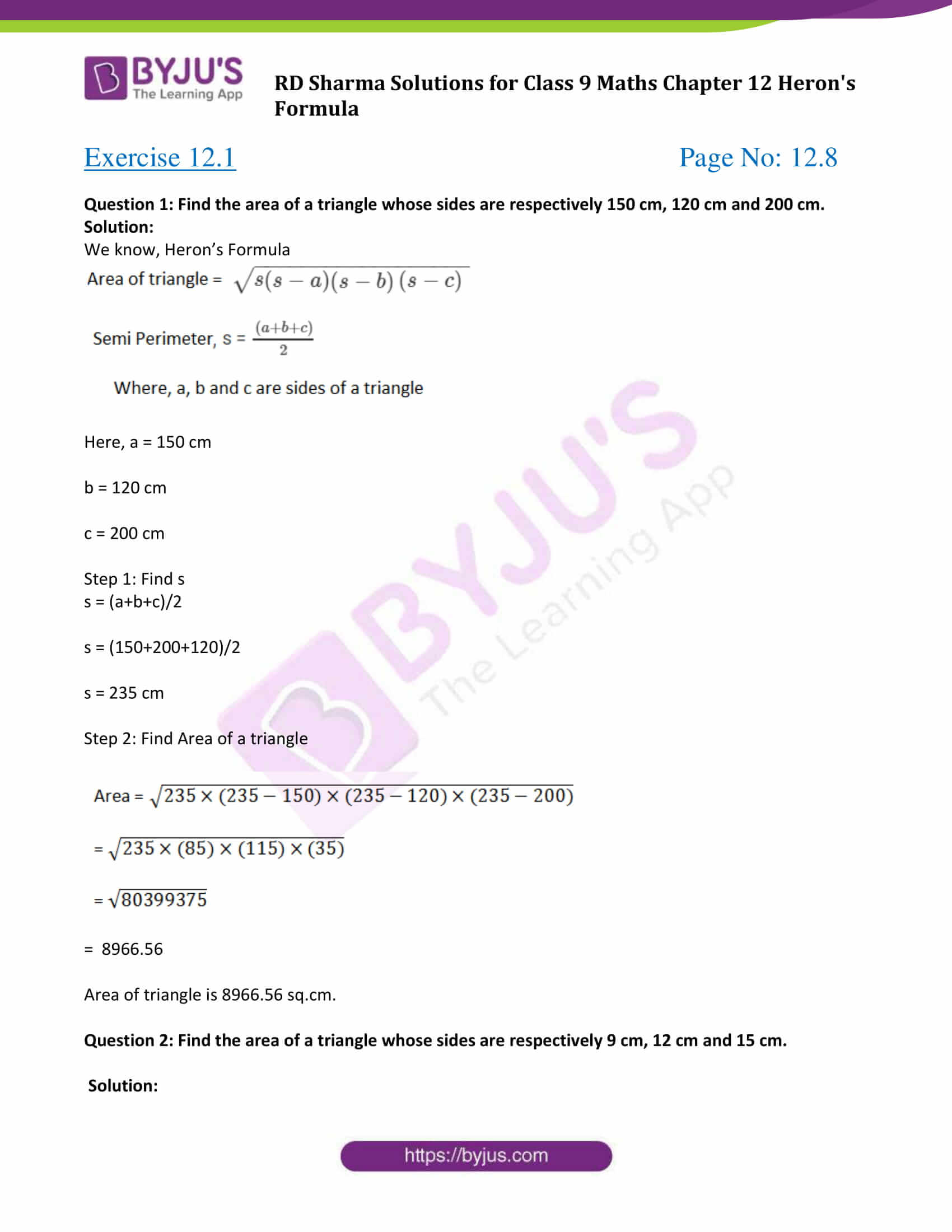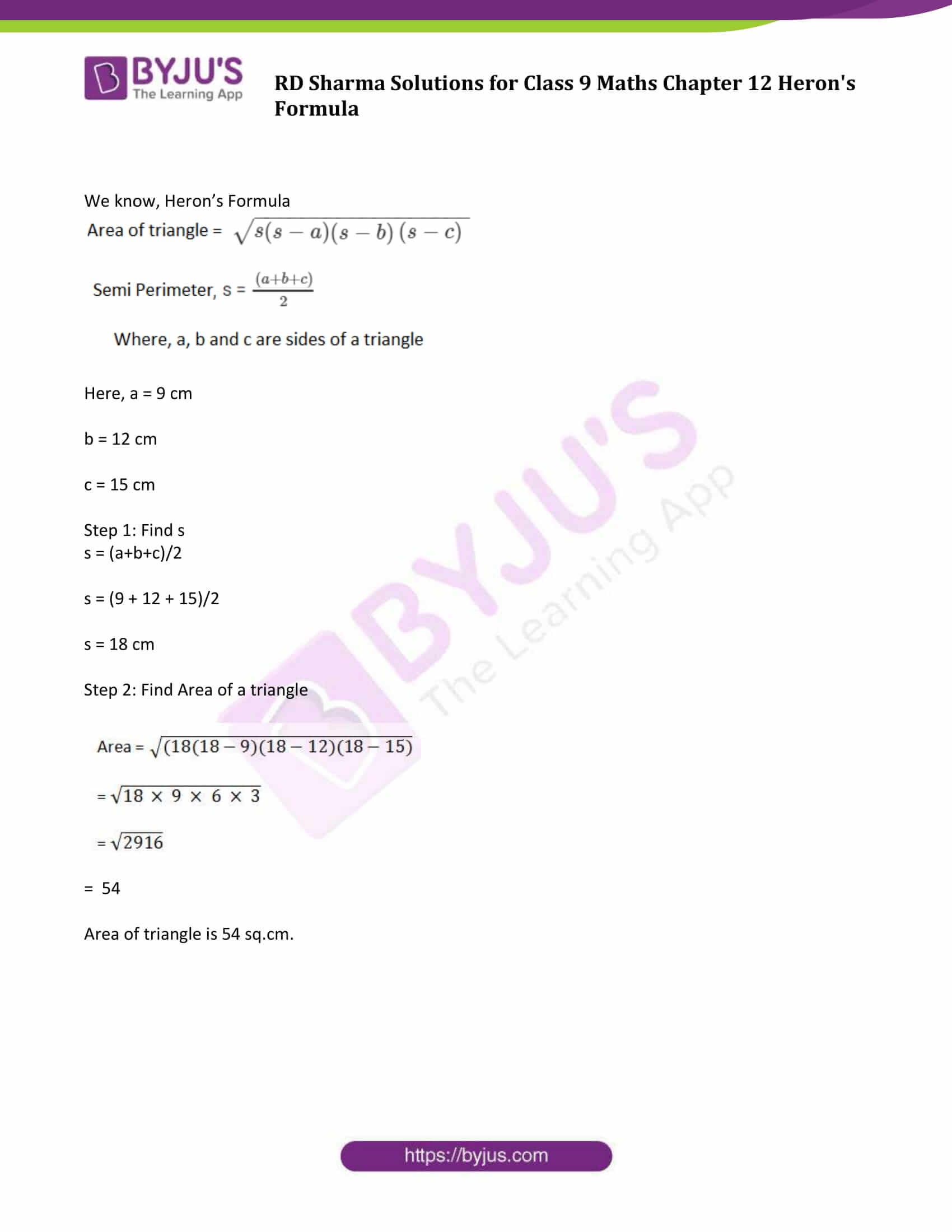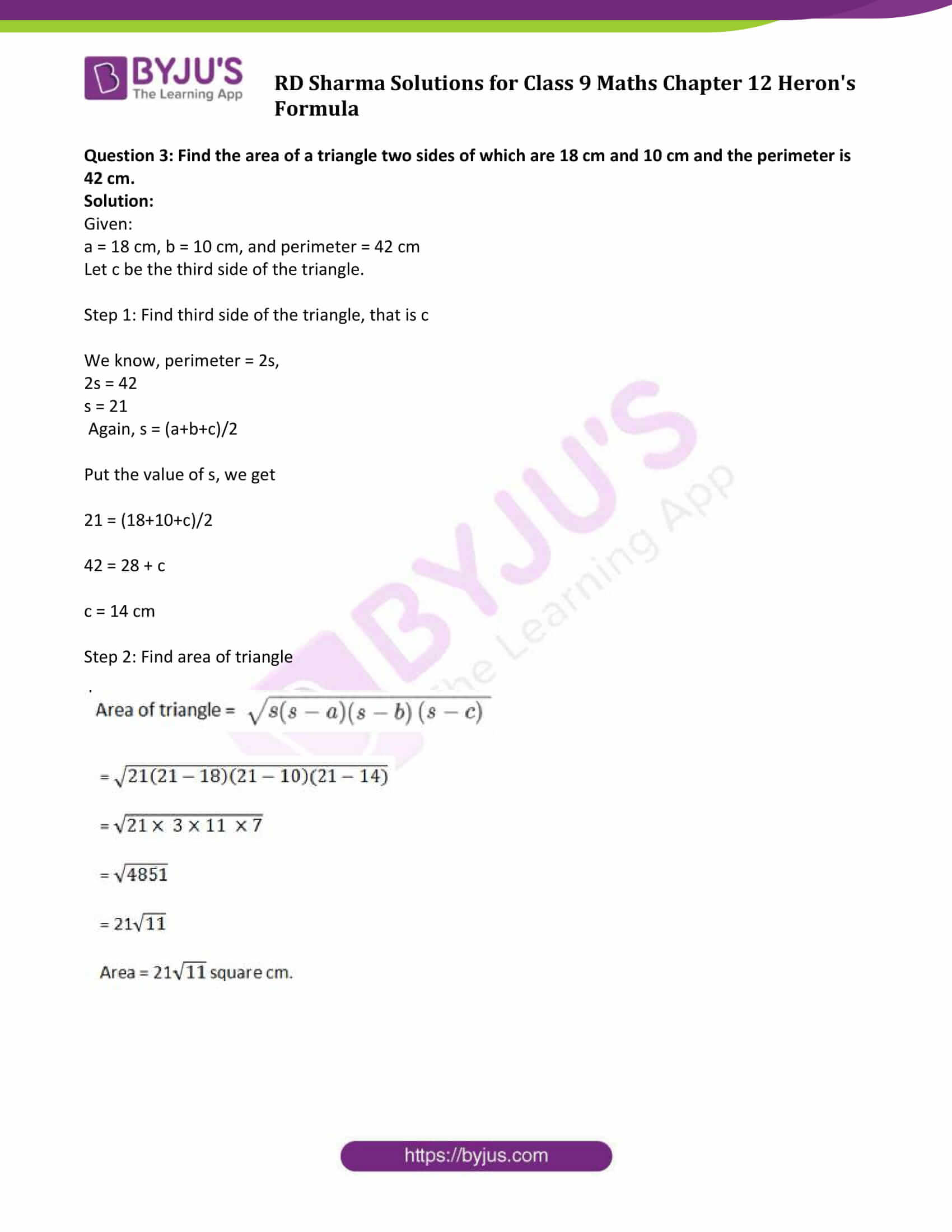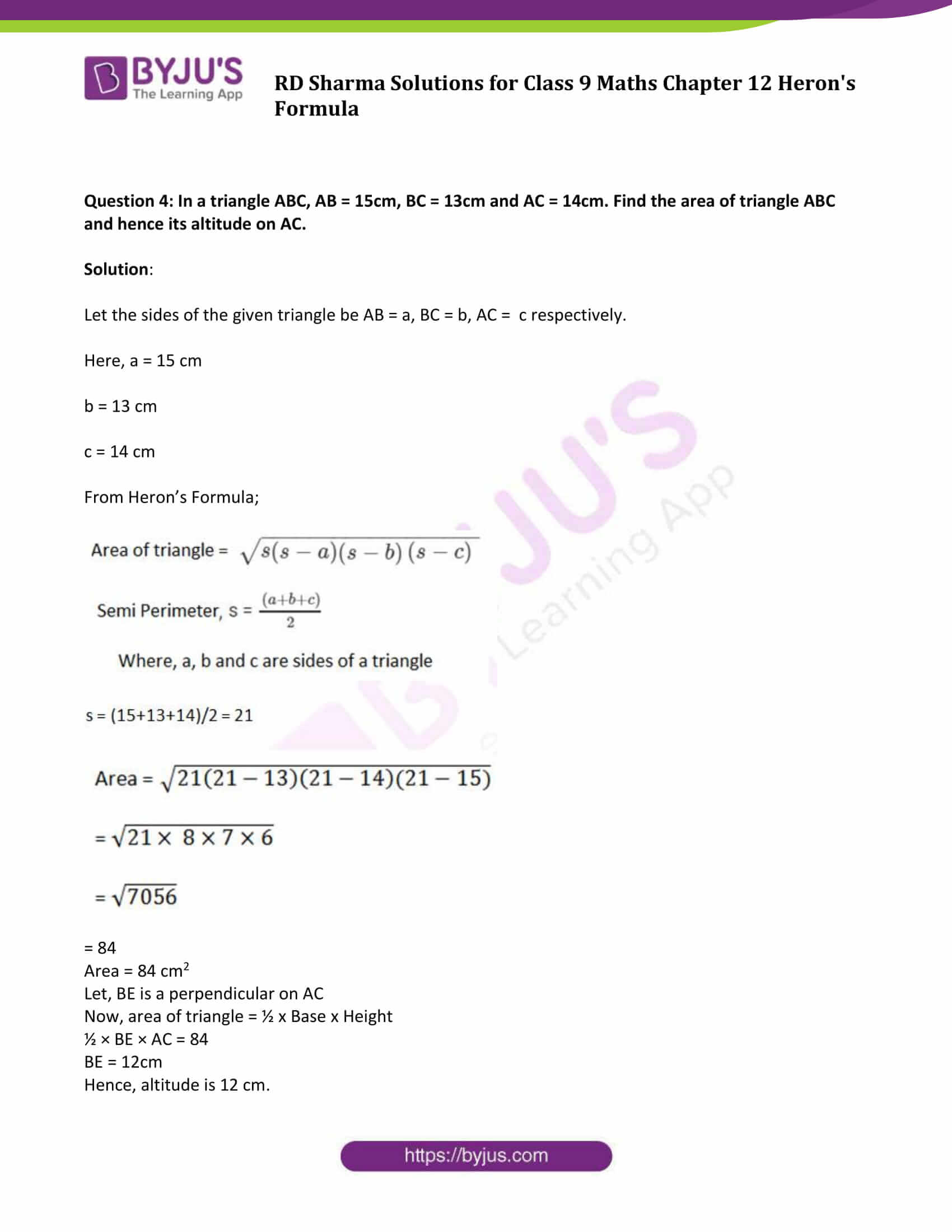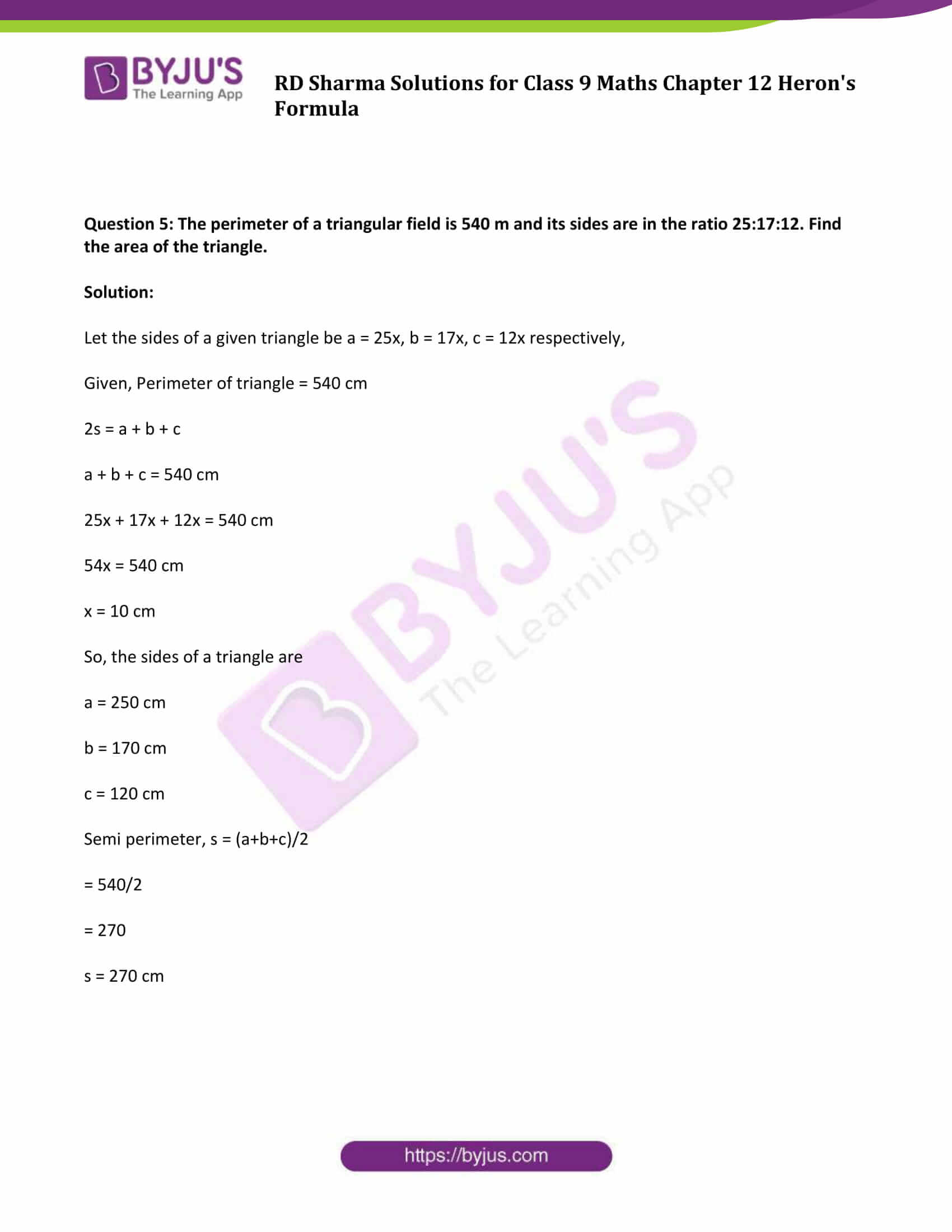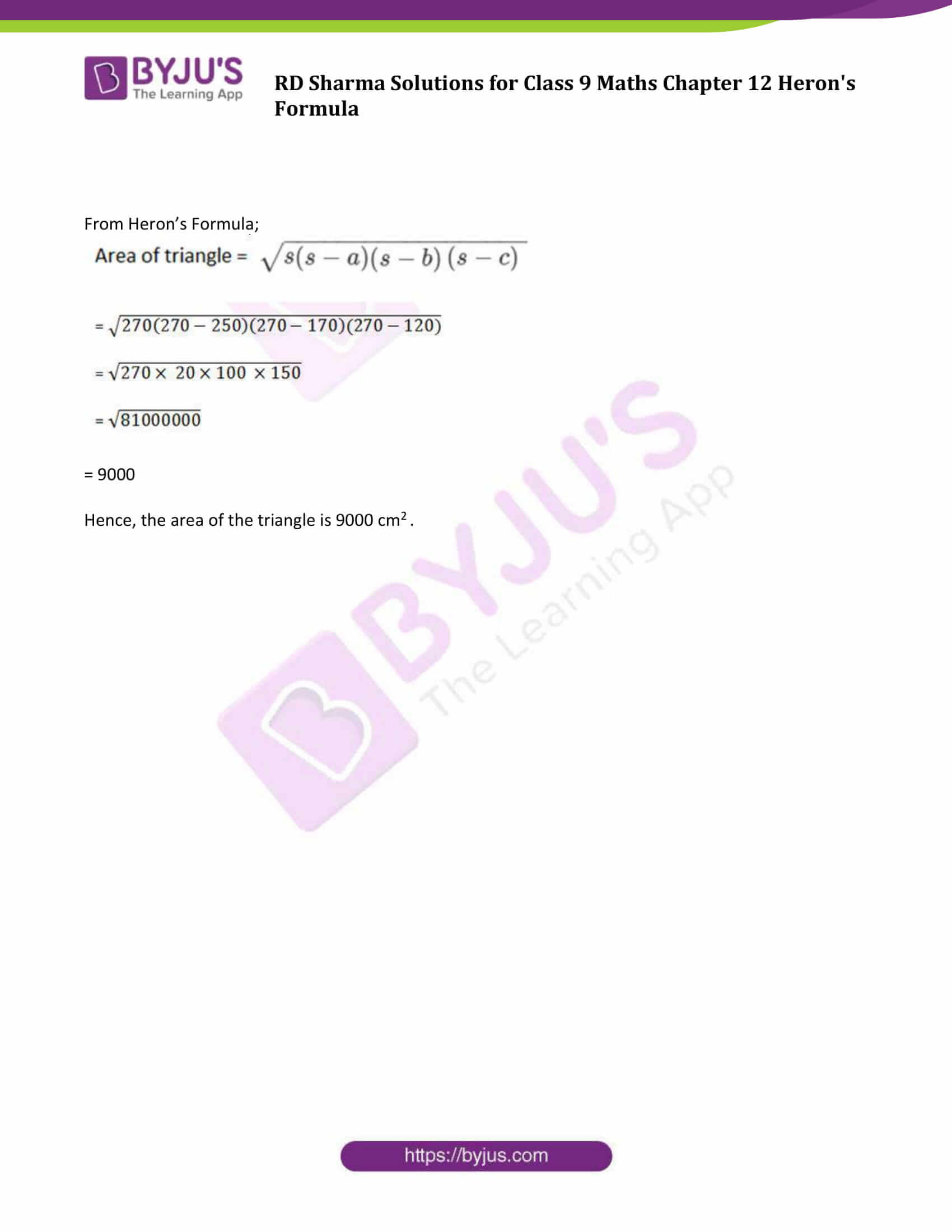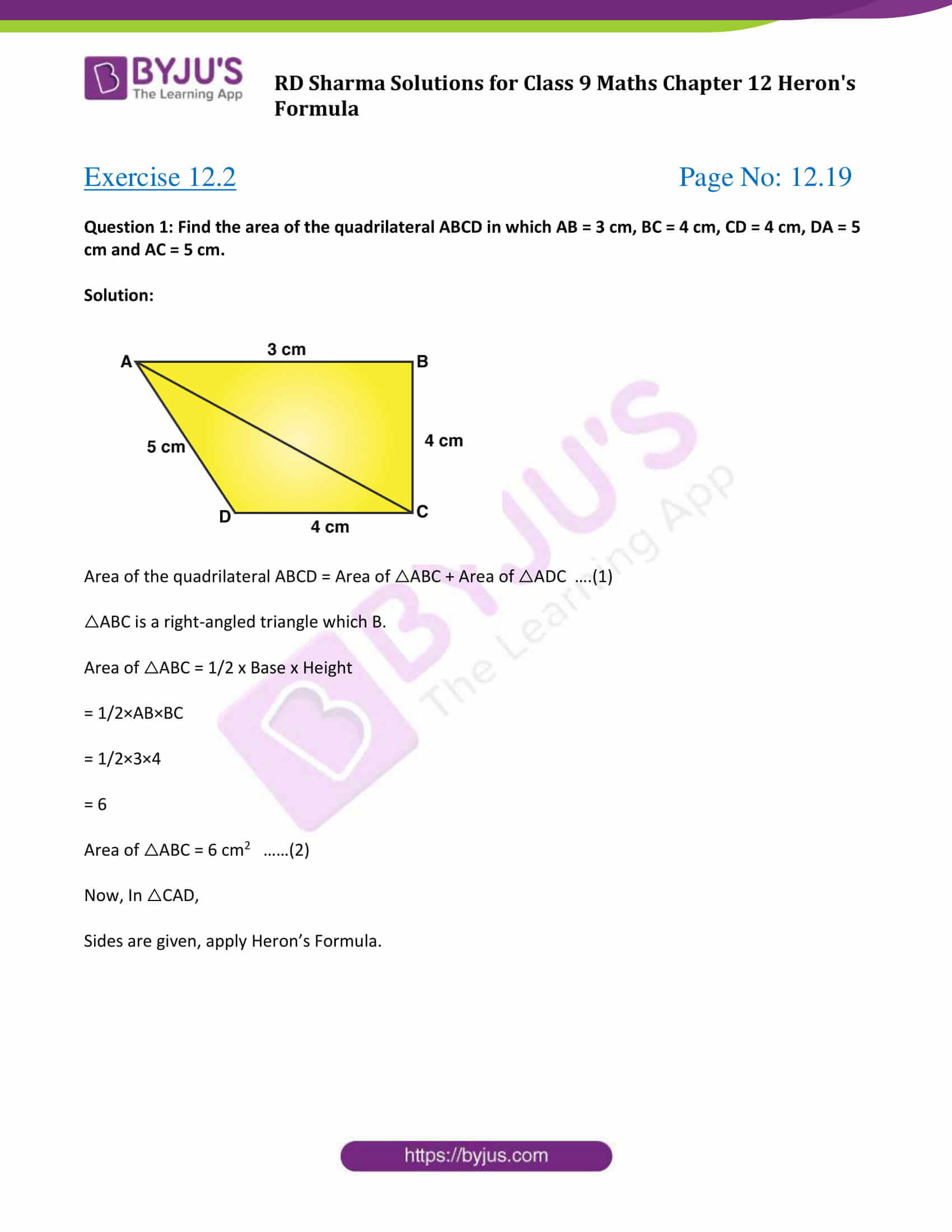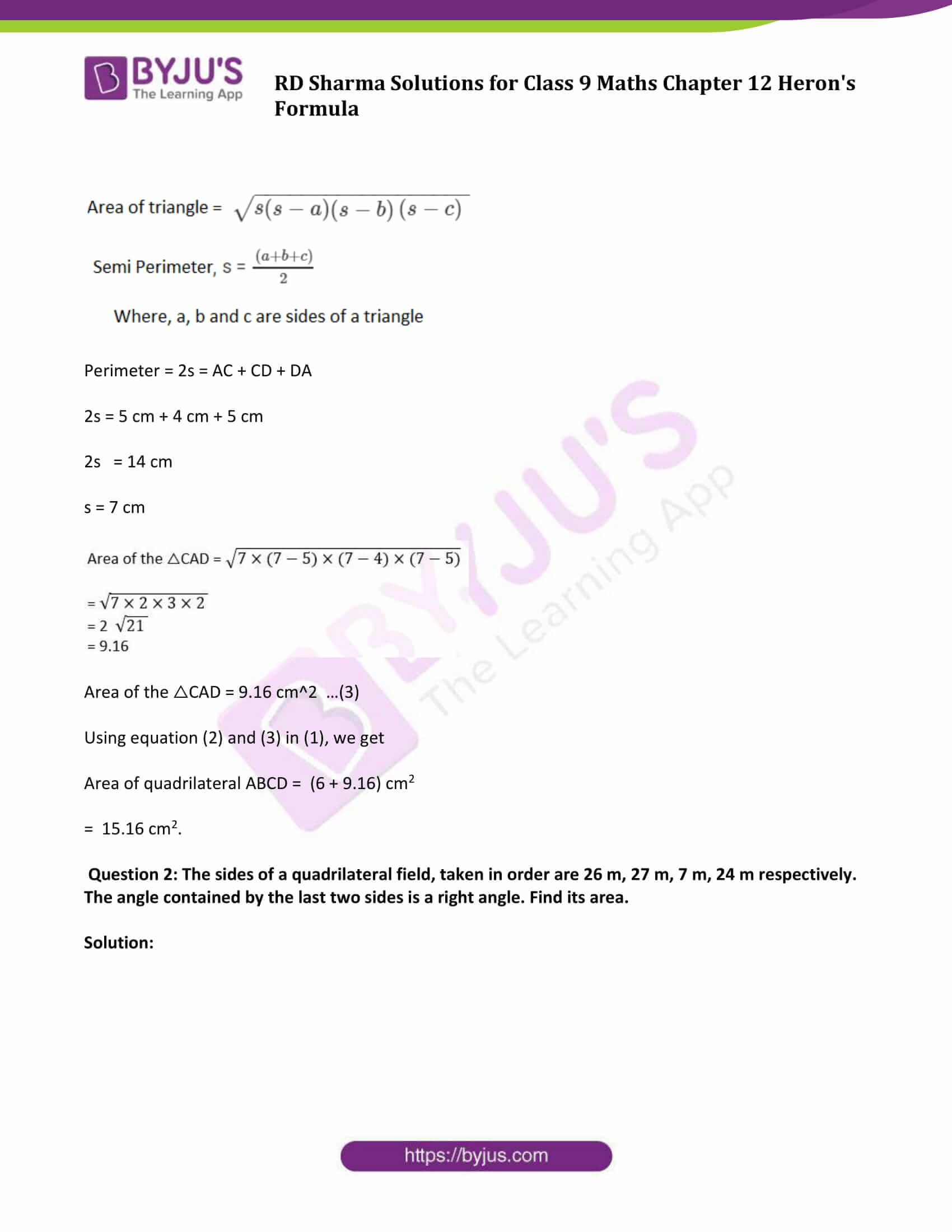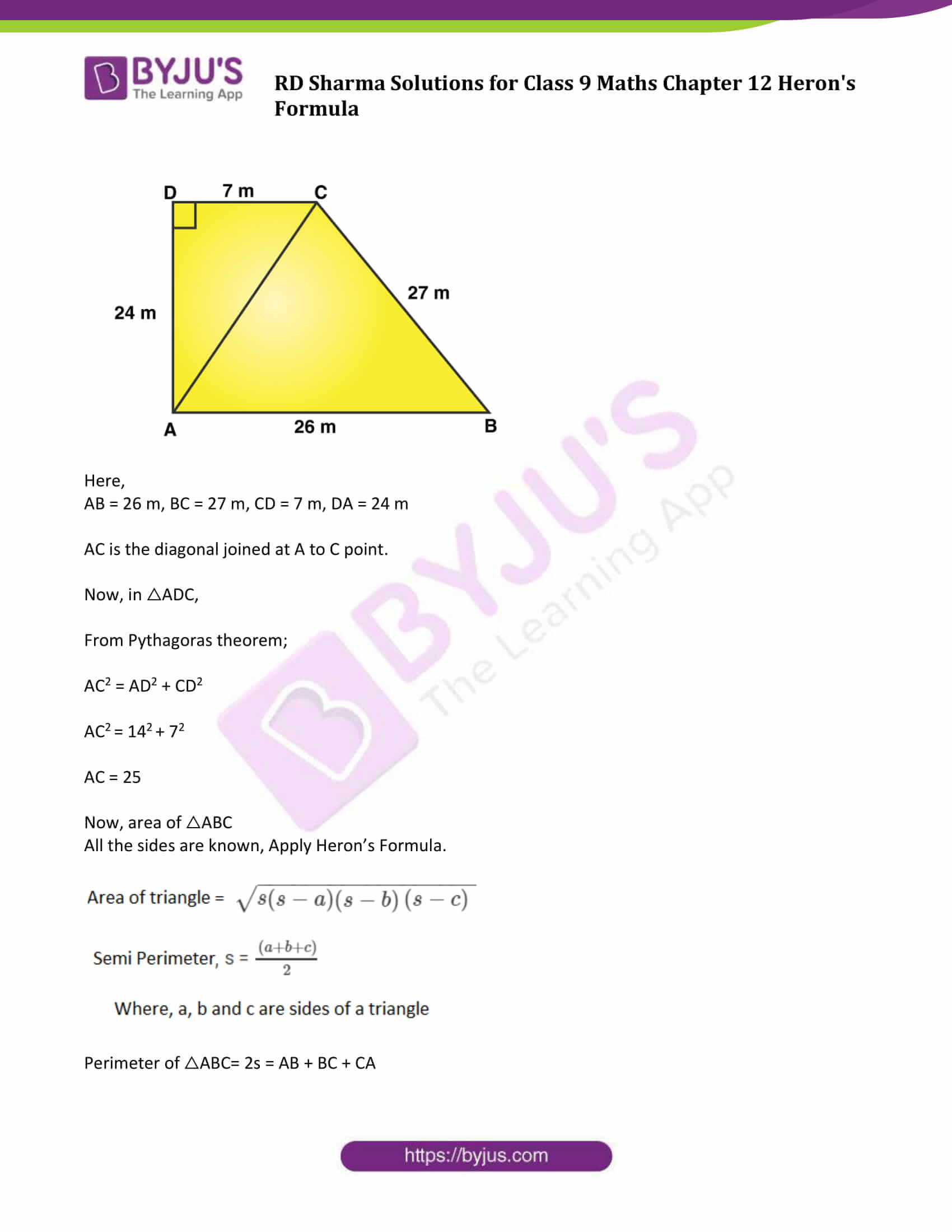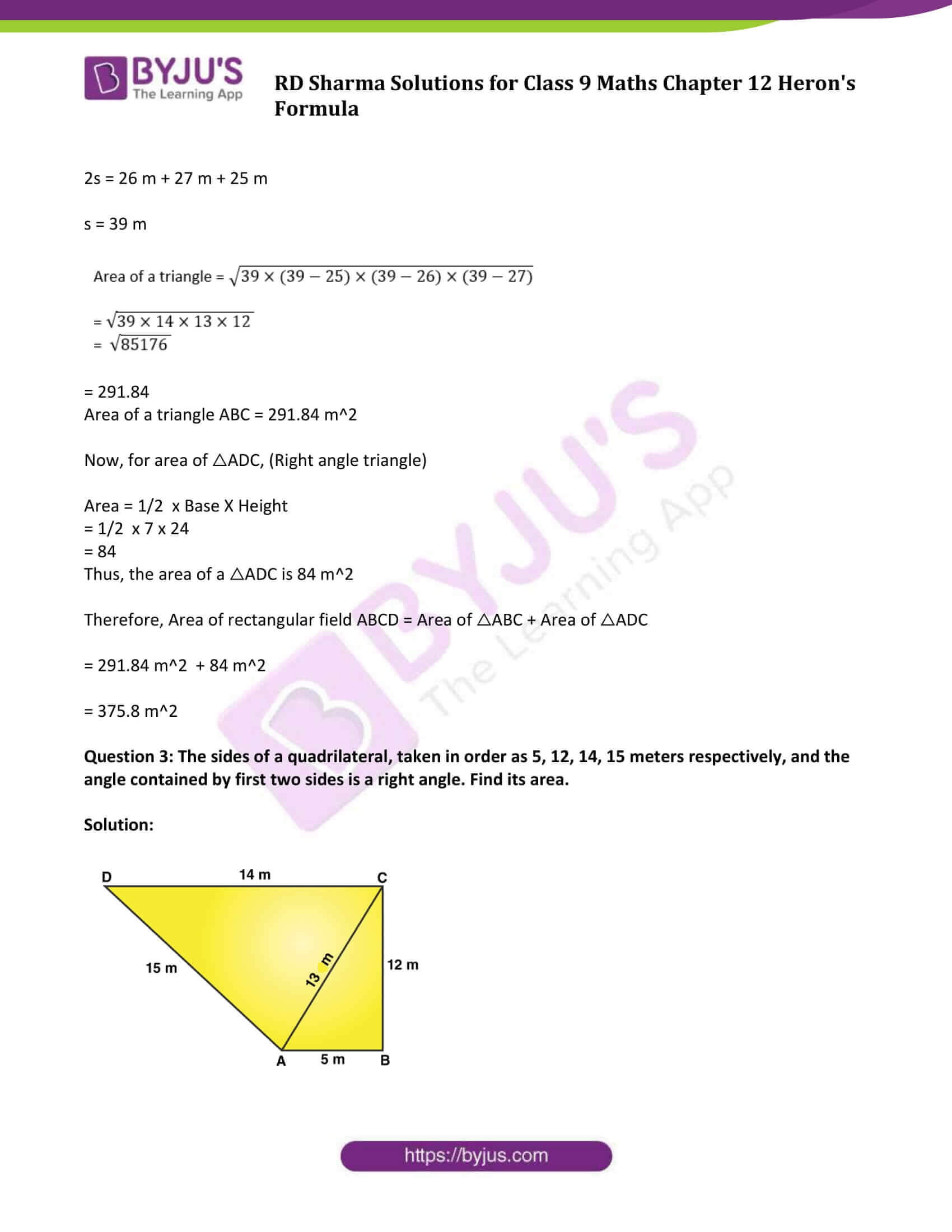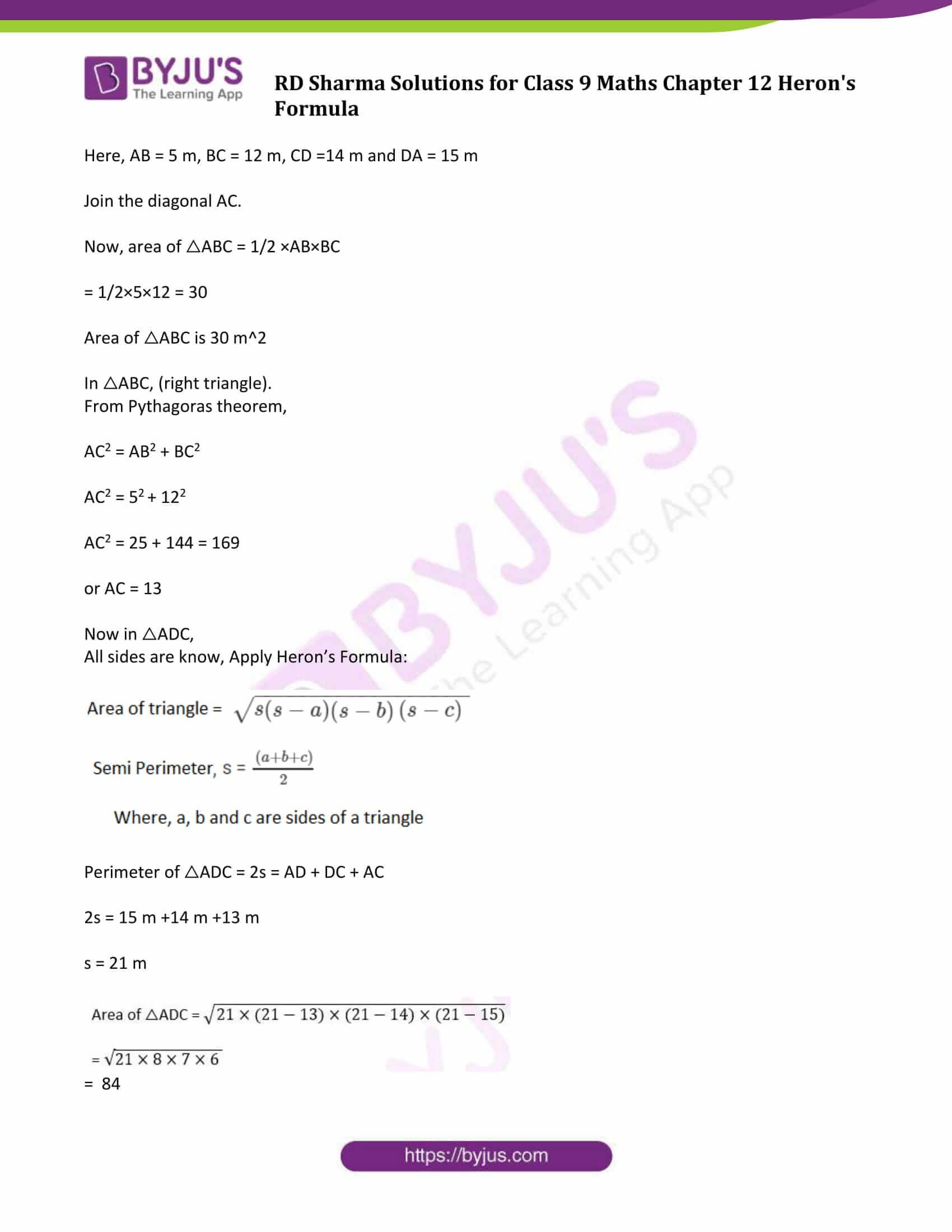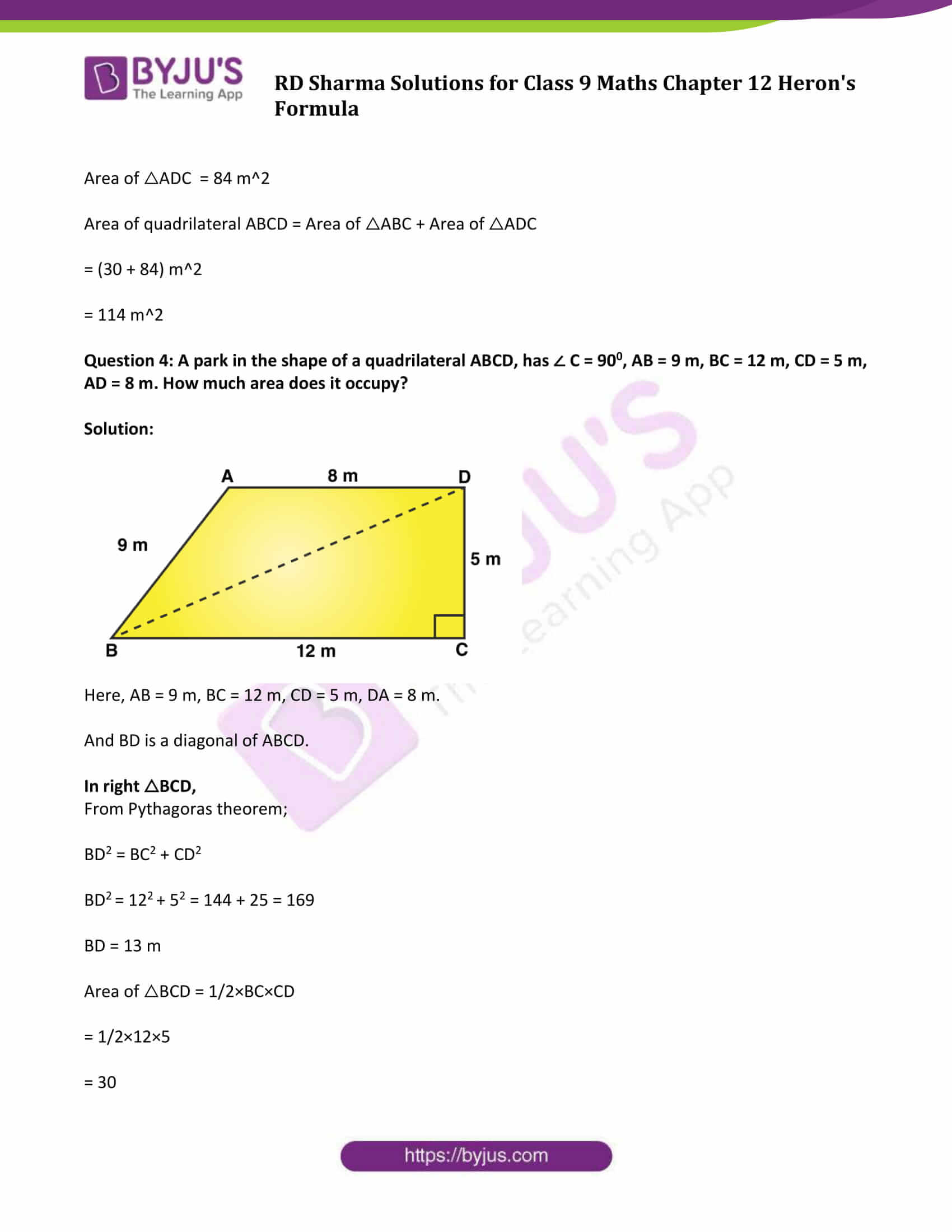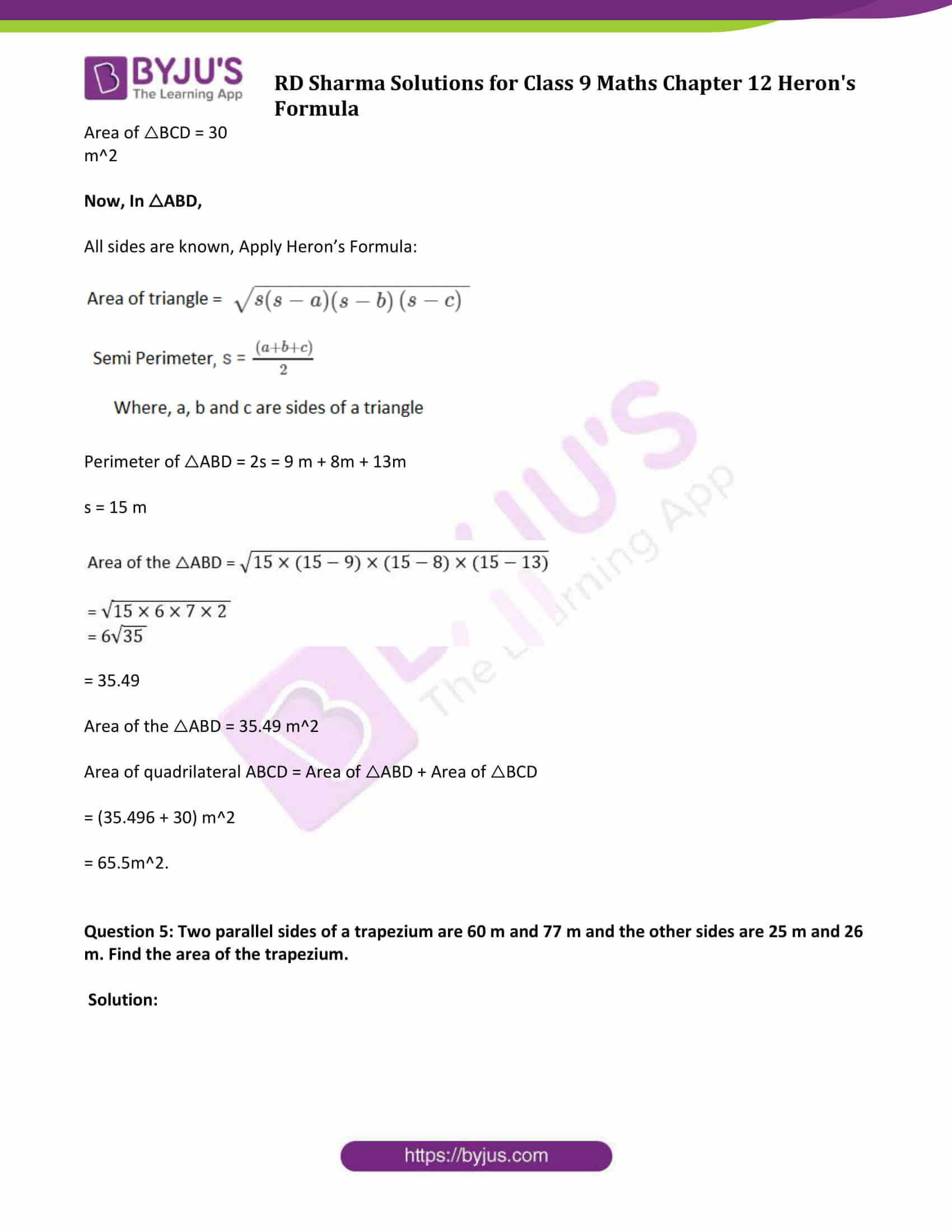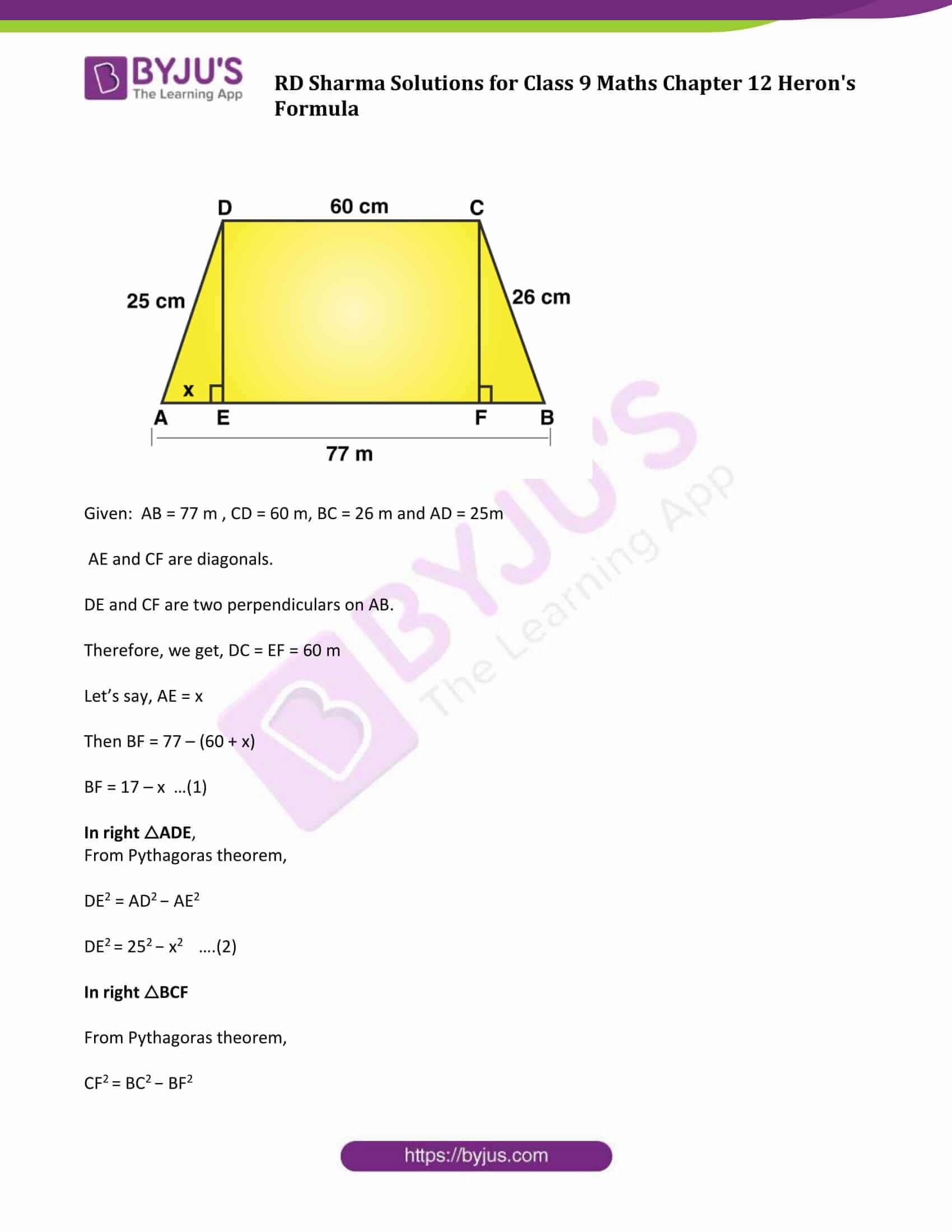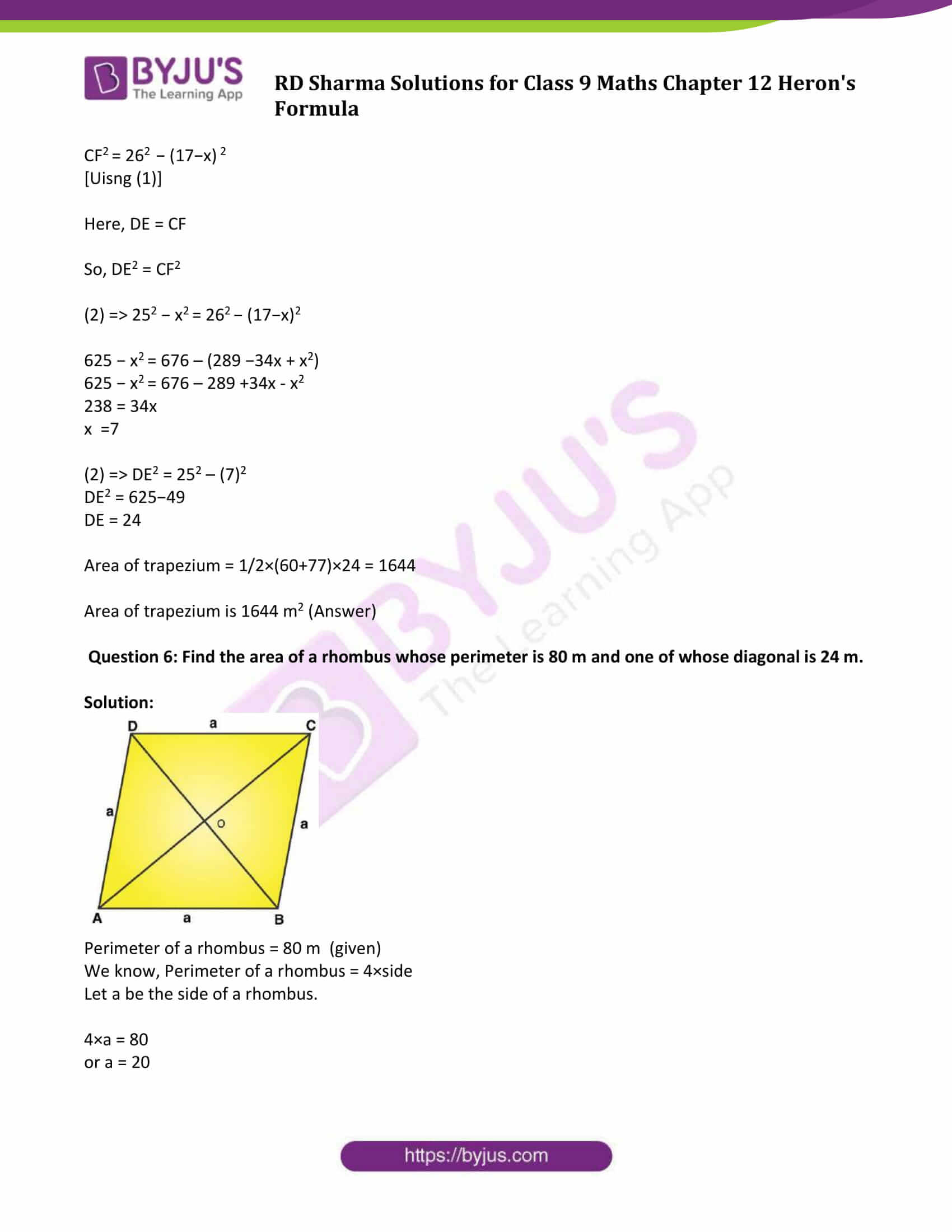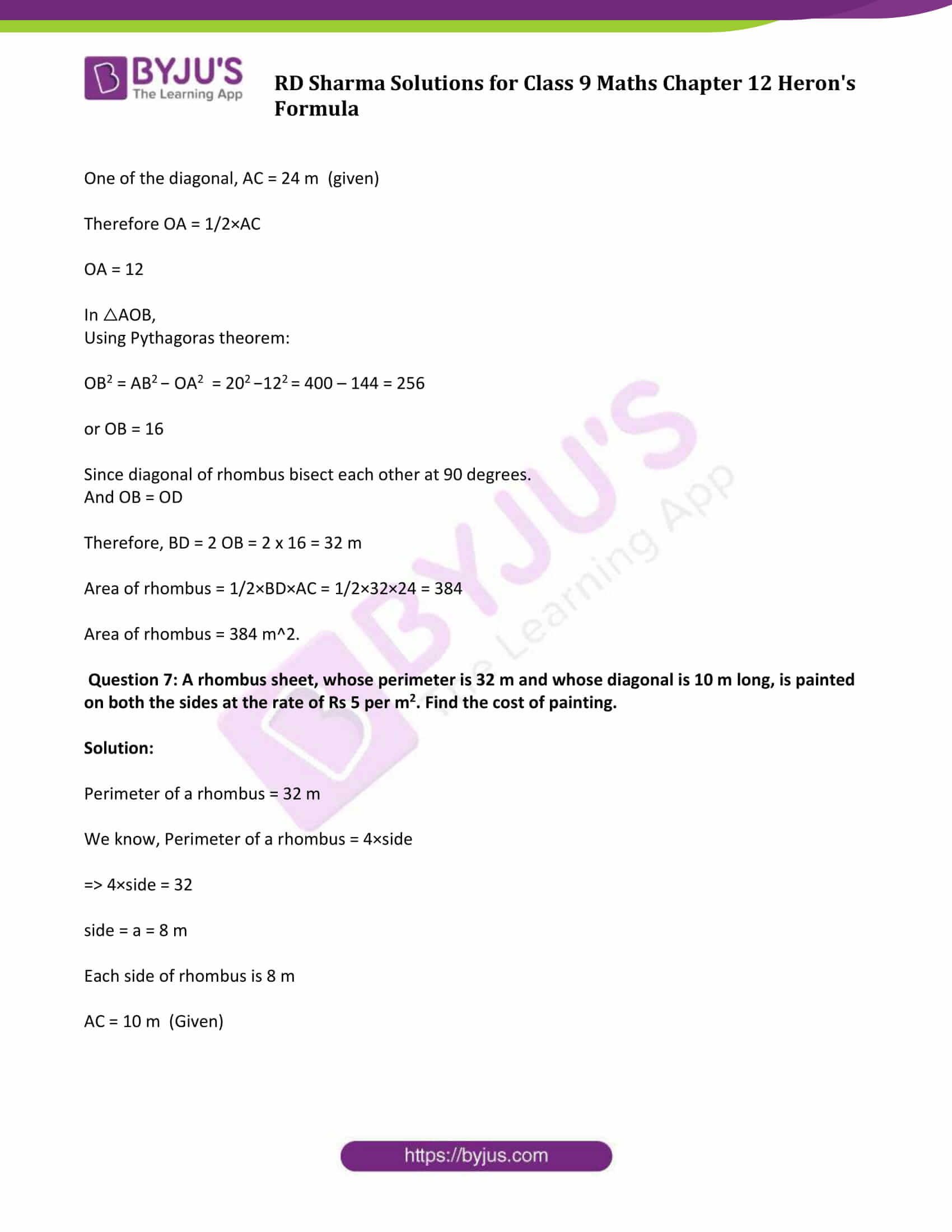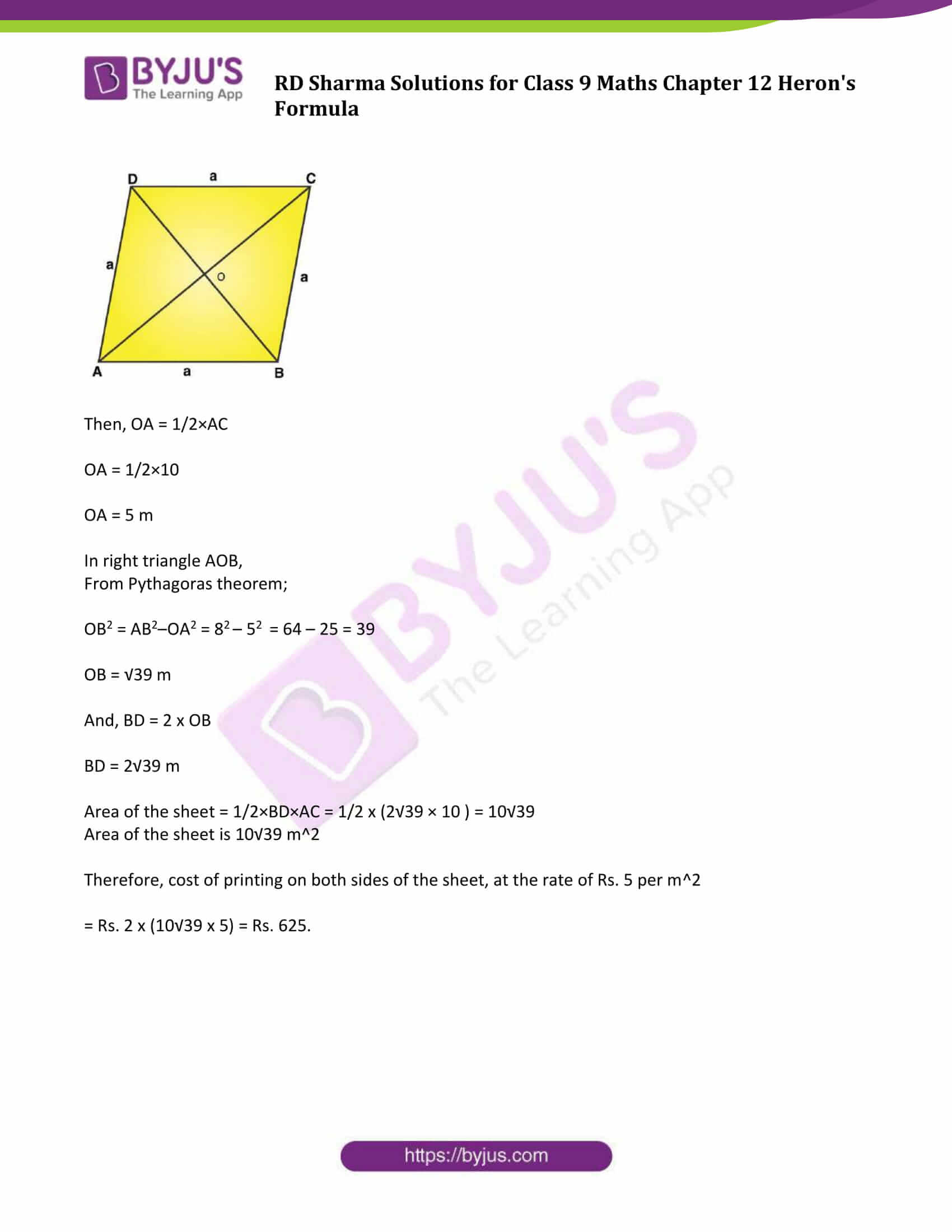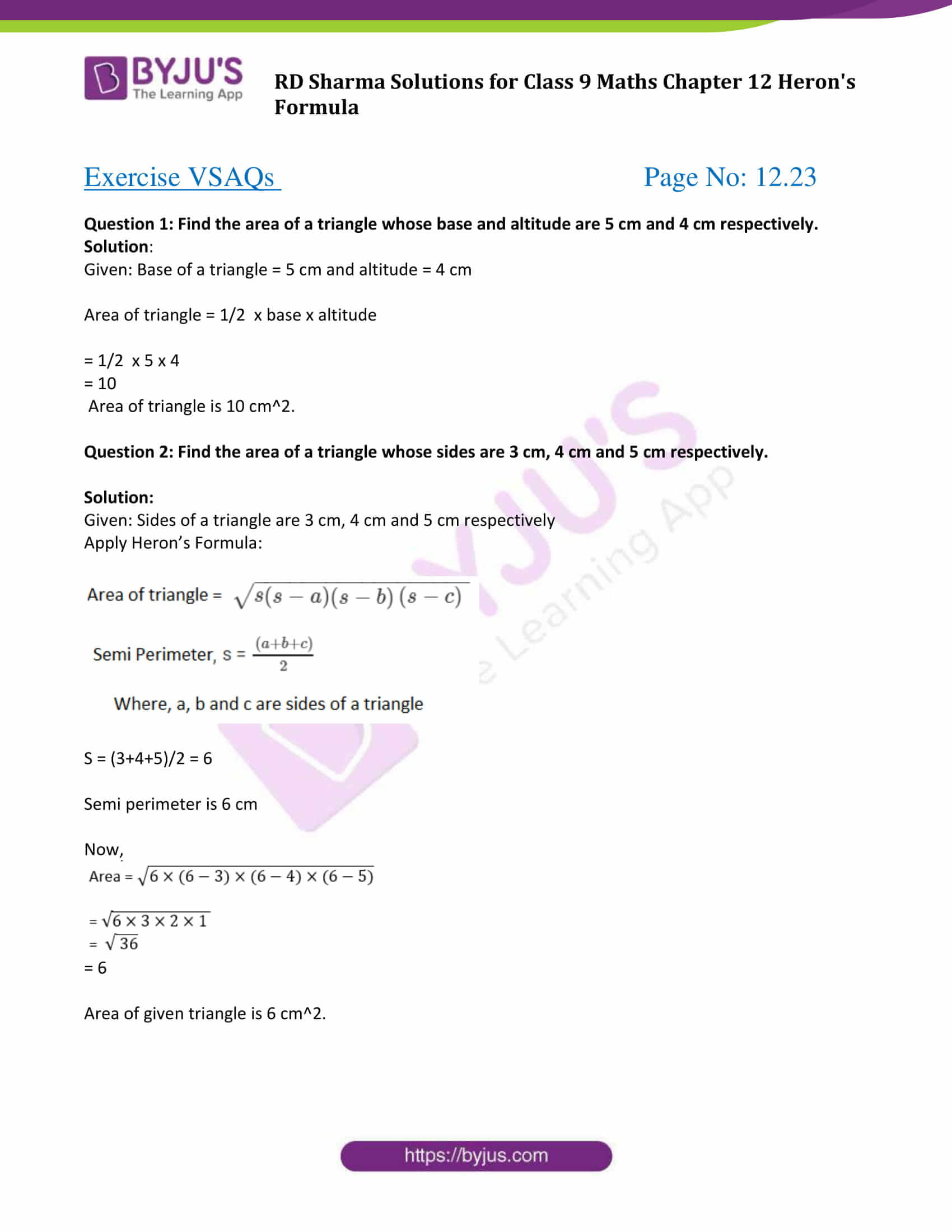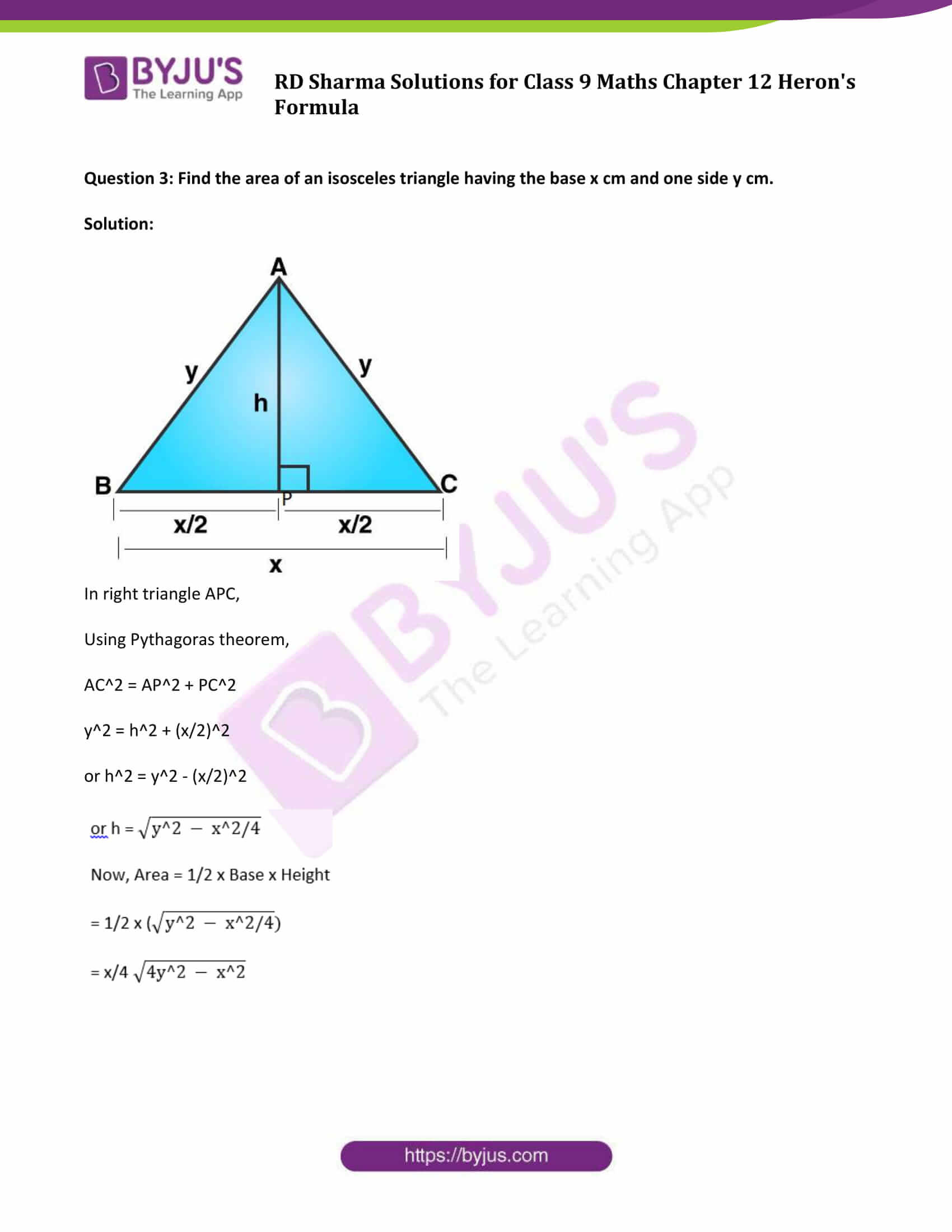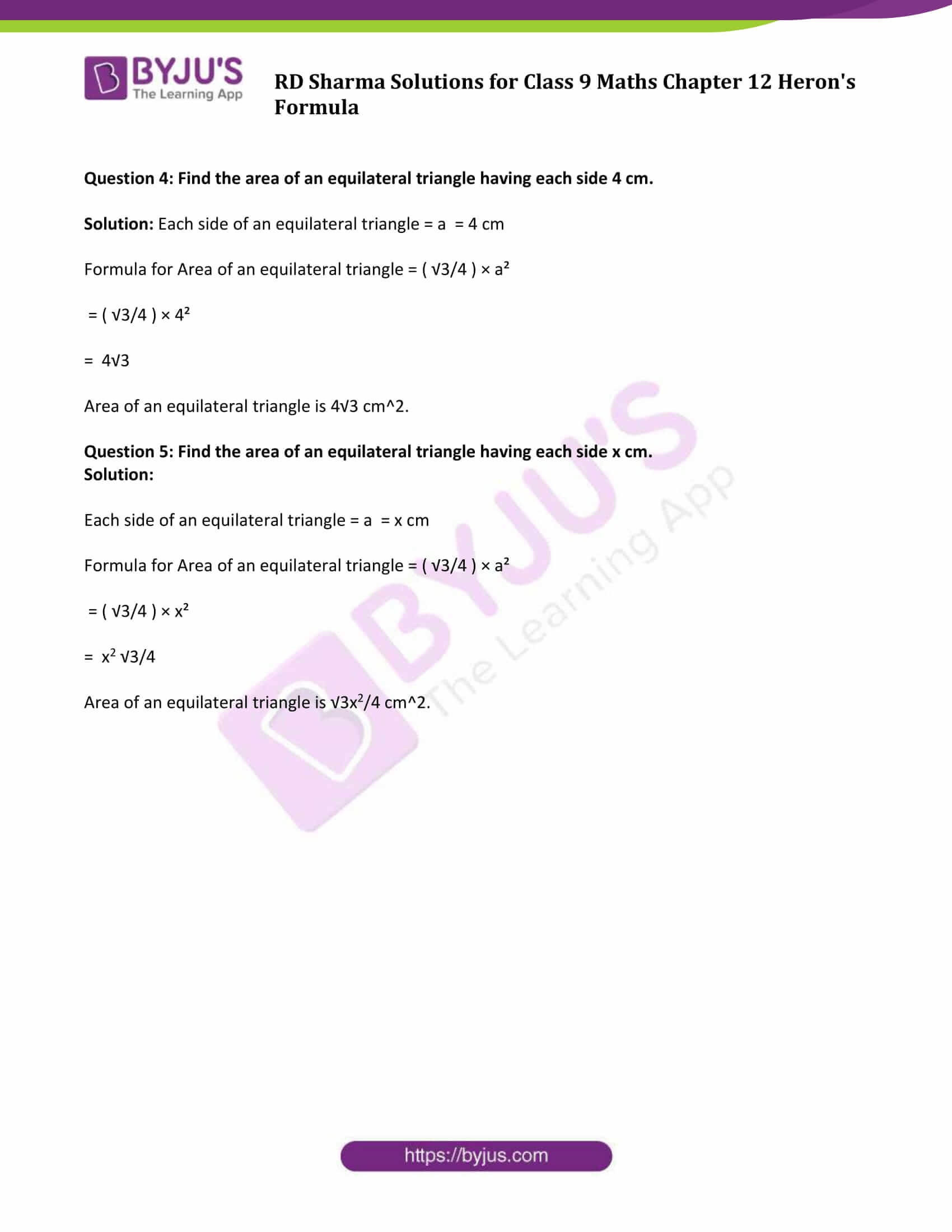### Exercise 12.1 Page No: 12.8

Question 1: Find the area of a triangle whose sides are respectively 150 cm, 120 cm and 200 cm.

Solution:

We know, Heron’s Formula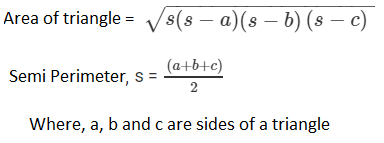Here, a = 150 cm

b = 120 cm

c = 200 cm

Step 1: Find s

s = (a+b+c)/2

s = (150+200+120)/2

s = 235 cm

Step 2: Find Area of a triangle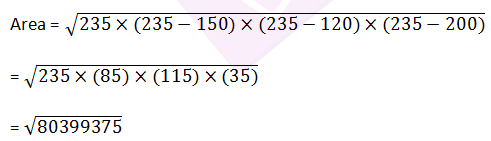= 8966.56

Area of triangle is 8966.56 sq.cm.

Question 2: Find the area of a triangle whose sides are respectively 9 cm, 12 cm and 15 cm.

Solution:

We know, Heron’s FormulaHere, a = 9 cm

b = 12 cm

c = 15 cm

Step 1: Find s

s = (a+b+c)/2

s = (9 + 12 + 15)/2

s = 18 cm

Step 2: Find Area of a triangle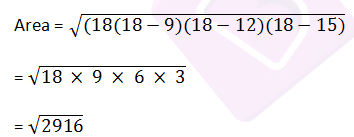= 54

Area of triangle is 54 sq.cm.

Question 3: Find the area of a triangle two sides of which are 18 cm and 10 cm and the perimeter is 42 cm.

Solution:

Given:

a = 18 cm, b = 10 cm, and perimeter = 42 cm

Let c be the third side of the triangle.

Step 1: Find third side of the triangle, that is c

We know, perimeter = 2s,

2s = 42

s = 21

Again, s = (a+b+c)/2

Put the value of s, we get

21 = (18+10+c)/2

42 = 28 + c

c = 14 cm

Step 2: Find area of triangle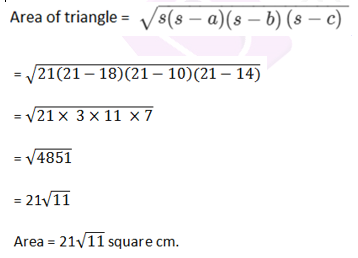Question 4: In a triangle ABC, AB = 15cm, BC = 13cm and AC = 14cm. Find the area of triangle ABC and hence its altitude on AC.

Solution:

Let the sides of the given triangle be AB = a, BC = b, AC = c respectively.

Here, a = 15 cm

b = 13 cm

c = 14 cm

From Heron’s Formula;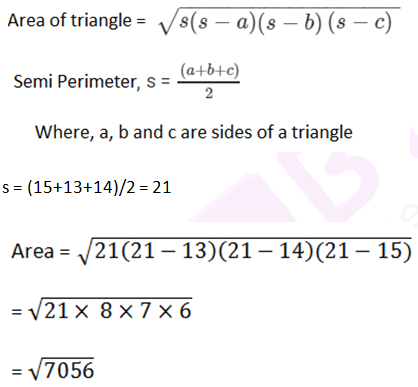= 84

Area = 84 cm2

Let, BE is a perpendicular on AC

Now, area of triangle = ½ x Base x Height

½ × BE × AC = 84

BE = 12cm

Hence, altitude is 12 cm.

Question 5: The perimeter of a triangular field is 540 m and its sides are in the ratio 25:17:12. Find the area of the triangle.

Solution:

Let the sides of a given triangle be a = 25x, b = 17x, c = 12x respectively,

Given, Perimeter of triangle = 540 cm

2s = a + b + c

a + b + c = 540 cm

25x + 17x + 12x = 540 cm

54x = 540 cm

x = 10 cm

So, the sides of a triangle are

a = 250 cm

b = 170 cm

c = 120 cm

Semi perimeter, s = (a+b+c)/2

= 540/2

= 270

s = 270 cm

From Heron’s Formula;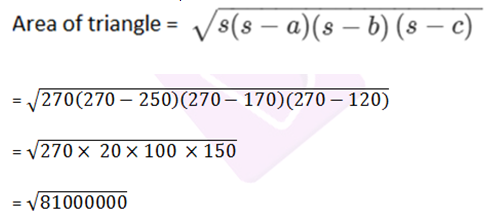= 9000

Hence, the area of the triangle is 9000 cm2 .

### Exercise 12.2 Page No: 12.19

Question 1: Find the area of the quadrilateral ABCD in which AB = 3 cm, BC = 4 cm, CD = 4 cm, DA = 5 cm and AC = 5 cm.

Solution: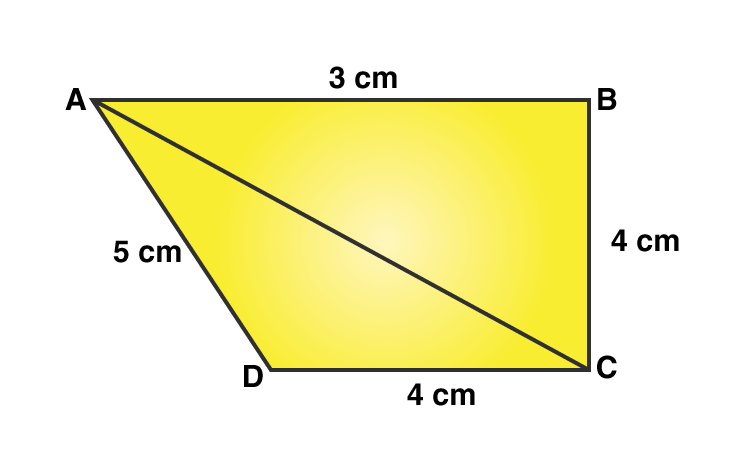Area of the quadrilateral ABCD = Area of △ABC + Area of △ADC ….(1)

△ABC is a right-angled triangle which B.

Area of △ABC = 1/2 x Base x Height

= 1/2×AB×BC

= 1/2×3×4

= 6

Area of △ABC = 6 cm2 ……(2)

Sides are given, apply Heron’s Formula.Perimeter = 2s = AC + CD + DA

2s = 5 cm + 4 cm + 5 cm

2s = 14 cm

s = 7 cm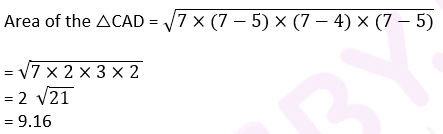Area of the △CAD = 9.16 cm2 …(3)

Using equation (2) and (3) in (1), we get

Area of quadrilateral ABCD = (6 + 9.16) cm2

= 15.16 cm2.

Question 2: The sides of a quadrilateral field, taken in order are 26 m, 27 m, 7 m, 24 m respectively. The angle contained by the last two sides is a right angle. Find its area.

Solution: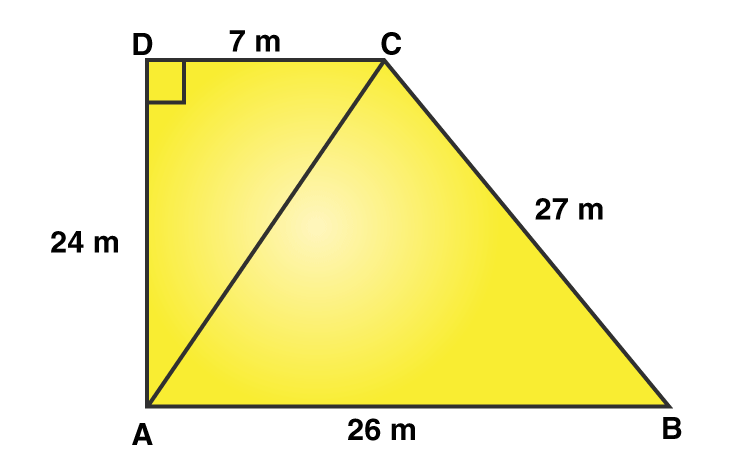Here,

AB = 26 m, BC = 27 m, CD = 7 m, DA = 24 m

AC is the diagonal joined at A to C point.

From Pythagoras theorem;

AC2 = 142 + 72

AC = 25

Now, area of △ABC

All the sides are known, Apply Heron’s Formula.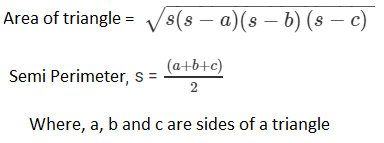Perimeter of △ABC= 2s = AB + BC + CA

2s = 26 m + 27 m + 25 m

s = 39 m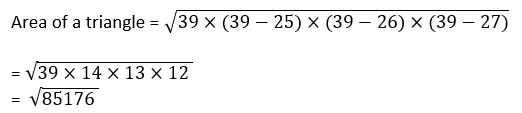= 291.84

Area of a triangle ABC = 291.84 m2

Now, for area of △ADC, (Right angle triangle)

Area = 1/2 x Base X Height

= 1/2 x 7 x 24

= 84

Thus, the area of a △ADC is 84 m2

Therefore, Area of rectangular field ABCD = Area of △ABC + Area of △ADC

= 291.84 m2 + 84 m2

= 375.8 m2

Question 3: The sides of a quadrilateral, taken in order as 5, 12, 14, 15 meters respectively, and the angle contained by first two sides is a right angle. Find its area.

Solution: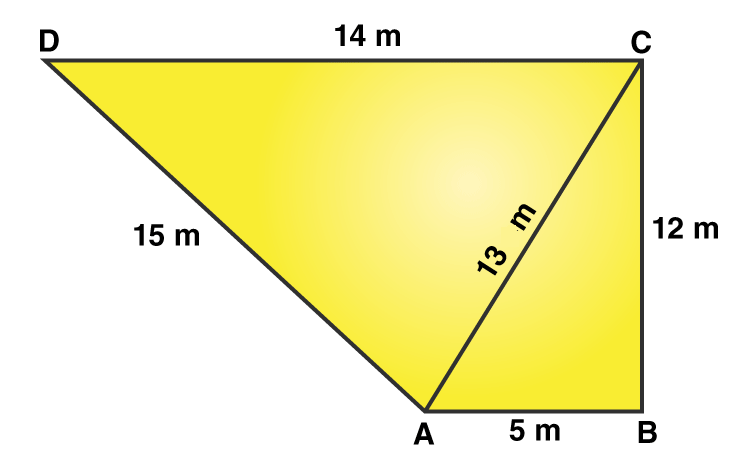Here, AB = 5 m, BC = 12 m, CD =14 m and DA = 15 m

Join the diagonal AC.

Now, area of △ABC = 1/2 ×AB×BC

= 1/2×5×12 = 30

Area of △ABC is 30 m2

In △ABC, (right triangle).

From Pythagoras theorem,

AC2 = AB2 + BC2

AC2 = 52 + 122

AC2 = 25 + 144 = 169

or AC = 13

All sides are know, Apply Heron’s Formula:2s = 15 m +14 m +13 m

s = 21 m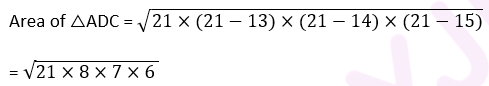= 84

Area of △ADC = 84 m2

Area of quadrilateral ABCD = Area of △ABC + Area of △ADC

= (30 + 84) m2

= 114 m2

Question 4: A park in the shape of a quadrilateral ABCD, has ∠ C = 900, AB = 9 m, BC = 12 m, CD = 5 m, AD = 8 m. How much area does it occupy?

Solution: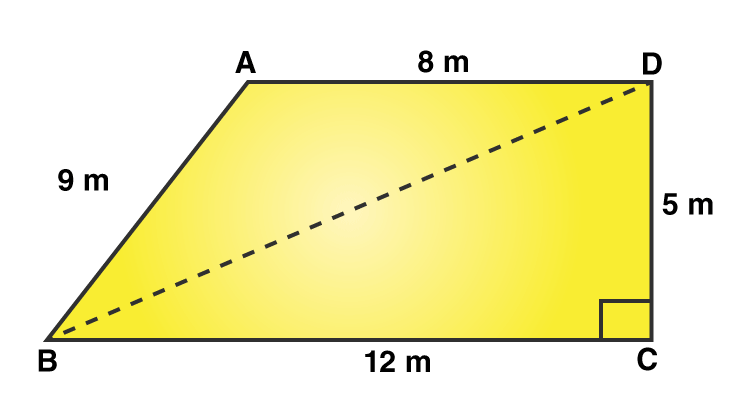Here, AB = 9 m, BC = 12 m, CD = 5 m, DA = 8 m.

And BD is a diagonal of ABCD.

In right △BCD,

From Pythagoras theorem;

BD2 = BC2 + CD2

BD2 = 122 + 52 = 144 + 25 = 169

BD = 13 m

Area of △BCD = 1/2×BC×CD

= 1/2×12×5

= 30

Area of △BCD = 30 m2

Now, In △ABD,

All sides are known, Apply Heron’s Formula:Perimeter of △ABD = 2s = 9 m + 8m + 13m

s = 15 m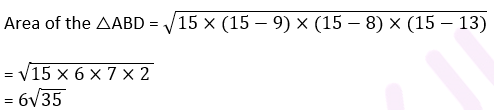= 35.49

Area of the △ABD = 35.49 m2

Area of quadrilateral ABCD = Area of △ABD + Area of △BCD

= (35.496 + 30) m2

= 65.5m2.

Question 5: Two parallel sides of a trapezium are 60 m and 77 m and the other sides are 25 m and 26 m. Find the area of the trapezium.

Solution: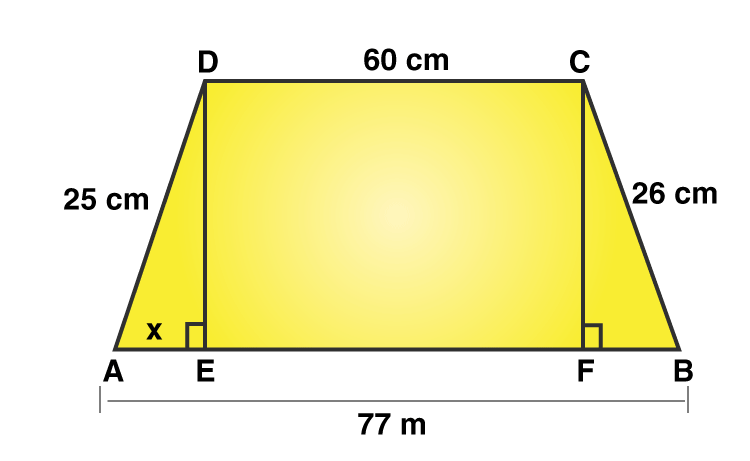Given: AB = 77 m , CD = 60 m, BC = 26 m and AD = 25m

AE and CF are diagonals.

DE and CF are two perpendiculars on AB.

Therefore, we get, DC = EF = 60 m

Let’s say, AE = x

Then BF = 77 – (60 + x)

BF = 17 – x …(1)

From Pythagoras theorem,

DE2 = 252 − x2 ….(2)

In right △BCF

From Pythagoras theorem,

CF2 = BC2 − BF2

CF2 = 262 − (17−x) 2

[Uisng (1)]

Here, DE = CF

So, DE2 = CF2

(2) ⇒ 252 − x2 = 262 − (17−x)2

625 − x2 = 676 – (289 −34x + x2)

625 − x2 = 676 – 289 +34x – x2

238 = 34x

x =7

(2) ⇒ DE2 = 252 – (7)2

DE2 = 625−49

DE = 24

Area of trapezium = 1/2×(60+77)×24 = 1644

Area of trapezium is 1644 m2 (Answer)

Question 6: Find the area of a rhombus whose perimeter is 80 m and one of whose diagonal is 24 m.

Solution: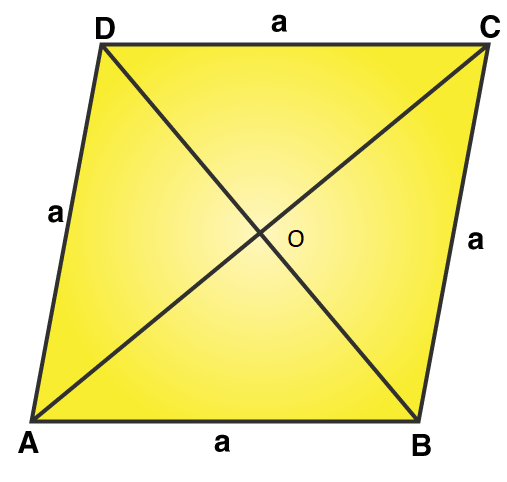Perimeter of a rhombus = 80 m (given)

We know, Perimeter of a rhombus = 4×side

Let a be the side of a rhombus.

4×a = 80

or a = 20

One of the diagonal, AC = 24 m (given)

Therefore OA = 1/2×AC

OA = 12

In △AOB,

Using Pythagoras theorem:

OB2 = AB2 − OA2 = 202 −122 = 400 – 144 = 256

or OB = 16

Since diagonal of rhombus bisect each other at 90 degrees.

And OB = OD

Therefore, BD = 2 OB = 2 x 16 = 32 m

Area of rhombus = 1/2×BD×AC = 1/2×32×24 = 384

Area of rhombus = 384 m2.

Question 7: A rhombus sheet, whose perimeter is 32 m and whose diagonal is 10 m long, is painted on both the sides at the rate of Rs 5 per m2. Find the cost of painting.

Solution:

Perimeter of a rhombus = 32 m

We know, Perimeter of a rhombus = 4×side

⇒ 4×side = 32

side = a = 8 m

Each side of rhombus is 8 m

AC = 10 m (Given)Then, OA = 1/2×AC

OA = 1/2×10

OA = 5 m

In right triangle AOB,

From Pythagoras theorem;

OB2 = AB2–OA2 = 82 – 52 = 64 – 25 = 39

OB = √39 m

And, BD = 2 x OB

BD = 2√39 m

Area of the sheet = 1/2×BD×AC = 1/2 x (2√39 × 10 ) = 10√39

Area of the sheet is 10√39 m2

Therefore, cost of printing on both sides of the sheet, at the rate of Rs. 5 per m2

= Rs. 2 x (10√39 x 5) = Rs. 625.

### Exercise VSAQs Page No: 12.23

Question 1: Find the area of a triangle whose base and altitude are 5 cm and 4 cm respectively.

Solution:

Given: Base of a triangle = 5 cm and altitude = 4 cm

Area of triangle = 1/2 x base x altitude

= 1/2 x 5 x 4

= 10

Area of triangle is 10 cm2.

Question 2: Find the area of a triangle whose sides are 3 cm, 4 cm and 5 cm respectively.

Solution:

Given: Sides of a triangle are 3 cm, 4 cm and 5 cm respectively

Apply Heron’s Formula:S = (3+4+5)/2 = 6

Semi perimeter is 6 cm

Now,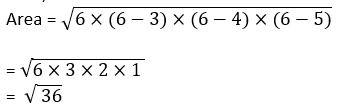= 6

Area of given triangle is 6 cm2.

Question 3: Find the area of an isosceles triangle having the base x cm and one side y cm.

Solution: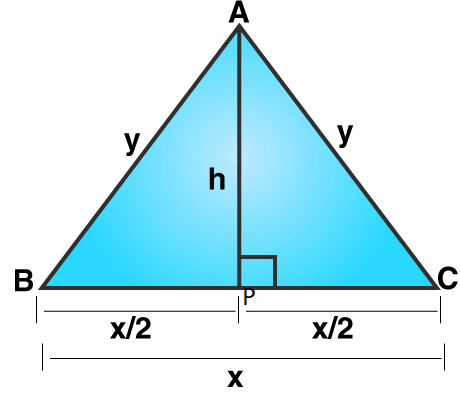In right triangle APC,

Using Pythagoras theorem,

AC2 = AP2 + PC2

y2 = h2 + (x/2)2

or h2 = y2 – (x/2)2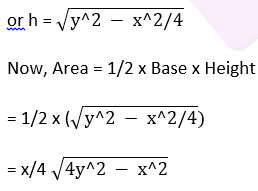Question 4: Find the area of an equilateral triangle having each side 4 cm.

Solution: Each side of an equilateral triangle = a = 4 cm

Formula for Area of an equilateral triangle = ( √3/4 ) × a²

= ( √3/4 ) × 4²

= 4√3

Area of an equilateral triangle is 4√3 cm2.

Question 5: Find the area of an equilateral triangle having each side x cm.

Solution:

Each side of an equilateral triangle = a = x cm

Formula for Area of an equilateral triangle = ( √3/4 ) × a²

= ( √3/4 ) × x²

= x2 √3/4

Area of an equilateral triangle is √3x2/4 cm2.

## RD Sharma Solutions for Class 9 Maths Chapter 12 Heron’s Formula

In the 12th Chapter of Class 9 RD Sharma Solutions students will study important concepts listed below:

• Heron’s Formula Introduction
• Applications of Heron’s Formula

Students can freely download all chapter solutions enlisted in the RD Sharma Class 9 textbook and prepare for examinations to score good marks.

## Frequently Asked Questions on RD Sharma Solutions for Class 9 Maths Chapter 12

### What is the main objective of RD Sharma Solutions for Class 9 Maths Chapter 12?

Students who want to intensify problem solving skills and score good marks are encouraged to follow RD Sharma Solutions created by expert faculty. These solutions in a clear format aim to help students to grasp the concepts in detail and clear their doubts instantly. Students can also cross check their answers while revising the problems of the textbook and know their level of preparation.

### Mention the key benefits of RD Sharma Solutions for Class 9 Maths Chapter 12

Following are the key benefits of RD Sharma Solutions for Class 9 Maths Chapter 12
1. Students can easily download the solutions for each exercise for a better understanding of concepts.
2. Graphs and illustrations are provided to help students in grasping the concepts with ease.
3. The solutions are formulated by highly knowledgeable teachers at BYJU’S in a comprehensive manner.

### Why should we practice RD Sharma Solutions for Class 9 Maths Chapter 12?

Students must practice RD Sharma Solutions for Class 9 Maths Chapter 12 to know the various methods of solving the problems with ease. These solutions also offer better conceptual knowledge of concepts as per student’s understanding capacity. Solving the textbook problems using these solutions help students to secure high marks in exams.